# DAV Class 5 Maths Chapter 4 Worksheet 2 Solutions

The DAV Class 5 Maths Book Solutions Pdf and DAV Class 5 Maths Chapter 4 Worksheet 2 Solutions of Fractional Numbers offer comprehensive answers to textbook questions.

## DAV Class 5 Maths Ch 4 Worksheet 2 Solutions

Question 1.
Compare the fractions by cross multiplication method.
(a) $$\frac{1}{2}$$ and $$\frac{1}{4}$$
Solution:(b) $$\frac{3}{5}$$ and $$\frac{3}{4}$$
Solution: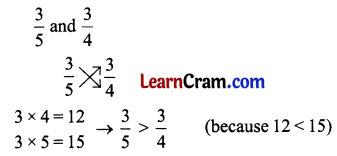(c) $$\frac{9}{10}$$ and $$\frac{2}{5}$$
Solution: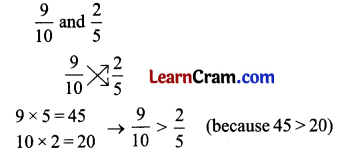(d) $$\frac{4}{9}$$ and $$\frac{5}{18}$$
Solution: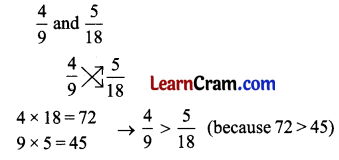(e) $$\frac{3}{5}$$ and $$\frac{3}{7}$$
Solution: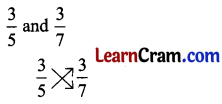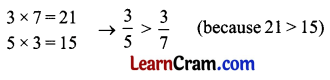(f) $$\frac{11}{18}$$ and $$\frac{1}{6}$$
Solution: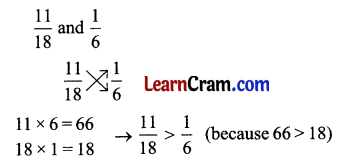Question 2.
Compare the fractions by taking the LCM.
(a) $$\frac{7}{2}$$ and $$\frac{5}{3}$$
Solution:
$$\frac{7}{2}$$ and $$\frac{5}{3}$$
LCM of 2 and 3 is 6
$$\frac{7}{2} \times \frac{3}{3}=\frac{21}{6}$$
$$\frac{5}{3} \times \frac{2}{2}=\frac{10}{6}$$
Now we compare like fractions
$$\frac{21}{6}$$ > $$\frac{10}{6}$$
∴ $$\frac{7}{6}$$ > $$\frac{5}{3}$$

(b) $$\frac{5}{6}$$ and $$\frac{3}{4}$$
Solution:
$$\frac{5}{6}$$ and $$\frac{3}{4}$$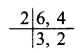2 × 3 × 3 = 12
LCM of 6 and 4 is 12
$$\frac{5}{6} \times \frac{2}{2}=\frac{10}{12}$$
$$\frac{3}{4} \times \frac{3}{3}=\frac{9}{12}$$
Now we compare like fractions
$$\frac{10}{12}$$ > $$\frac{9}{12}$$
∴ $$\frac{5}{6}$$ > $$\frac{3}{4}$$(c) $$\frac{1}{4}$$ and $$\frac{2}{5}$$
Solution:
$$\frac{1}{4}$$ and $$\frac{2}{5}$$
LCM of 5 and 5 is 20
$$\frac{1}{4} \times \frac{5}{5}=\frac{5}{20}$$
$$\frac{2}{5} \times \frac{4}{4}=\frac{8}{20}$$
Now we compare like fractions
$$\frac{5}{20}$$ < $$\frac{8}{20}$$
∴ $$\frac{1}{4}$$ < $$\frac{2}{5}$$

(d) $$\frac{3}{10}$$ and $$\frac{3}{4}$$
Solution:
$$\frac{3}{10}$$ and $$\frac{3}{4}$$2 × 5 × 2 = 20
LCM of 10 and 4 is 20
$$\frac{3}{10} \times \frac{2}{2}=\frac{6}{20}$$
$$\frac{3}{4} \times \frac{5}{5}=\frac{15}{20}$$
Now we compare like fractions
$$\frac{6}{20}$$ < $$\frac{15}{20}$$
∴ $$\frac{3}{10}$$ < $$\frac{3}{4}$$

(e) $$\frac{3}{5}$$ and $$\frac{5}{6}$$
Solution:
$$\frac{3}{5}$$ and $$\frac{5}{6}$$
LCM of 5 and 6 is 30
$$\frac{3}{5} \times \frac{6}{6}=\frac{18}{30}$$
$$\frac{5}{6} \times \frac{5}{5}=\frac{25}{30}$$
Now we compare like fractions
$$\frac{18}{30}$$ < $$\frac{25}{30}$$
∴ $$\frac{3}{5}$$ < $$\frac{5}{6}$$

(f) $$\frac{2}{9}$$ and $$\frac{3}{7}$$
Solution:
$$\frac{2}{9}$$ and $$\frac{3}{7}$$
LCM of 9 and 7 is 63
$$\frac{2}{9} \times \frac{7}{7}=\frac{14}{63}$$
$$\frac{3}{7} \times \frac{9}{9}=\frac{27}{63}$$
Now we compare like fractions
$$\frac{14}{63}$$ < $$\frac{27}{63}$$
∴ $$\frac{2}{9}$$ < $$\frac{3}{7}$$

Question 3.
Compare the following pairs of fractional numbers.
(a) $$5 \frac{1}{2}$$ and $$\frac{5}{2}$$
Solution:(b) $$\frac{9}{7}$$ and $$1 \frac{2}{7}$$
Solution: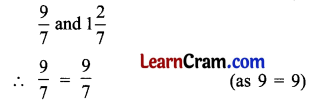(c) $$\frac{19}{7}$$ and $$2 \frac{1}{6}$$
Solution: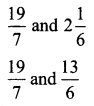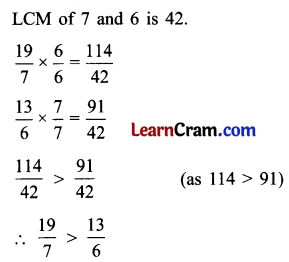(d) $$4 \frac{3}{4}$$ and $$\frac{20}{5}$$
Solution:(e) $$1 \frac{1}{2}$$ and $$\frac{5}{4}$$
Solution: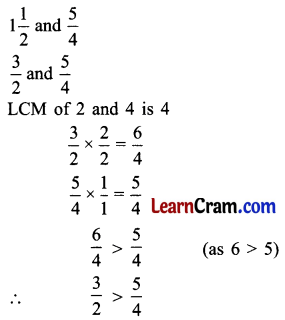(f) $$1 \frac{1}{5}$$ and $$\frac{5}{4}$$
Solution:DAV Class 5 Maths Chapter 4 Worksheet 2 Notes

In like fractions, the greater the numerator, the greater will be the value of the fractional number.
$$\frac{4}{7}>\frac{2}{7}$$

In Unlike fractions, with the same numerator, the greater the denominator, the smaller will be the value of the fractional numerator.
$$\frac{2}{10}<\frac{2}{8}$$

In case of $$\frac{3}{4}$$ and $$\frac{7}{8}$$
Method 1: First we convert the unlike fractions $$\frac{3}{4}$$ and $$\frac{7}{8}$$ into like fractions.
For that find the Lowest Common Multiple (LCM) of denominators i.e., 4 and 8.
$$\frac{3}{4} \times \frac{2}{2}=\frac{6}{8}$$
$$\frac{7}{8} \times \frac{1}{1}=\frac{7}{8}$$
Now compare like fractions $$\frac{6}{8}$$ and $$\frac{7}{8}$$
$$\frac{6}{8}<\frac{7}{8}$$ (because 7 > 6)

Method 2: Quick Method of Comparing.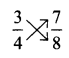cross multiplication
3 × 8 = 24
4 × 7 = 28
$$\frac{3}{4}<\frac{7}{8}$$ (because 24 < 28)